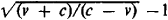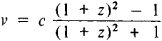# red shift

(redirected from red shifts)
Also found in: Dictionary, Thesaurus.
Related to red shifts: Redshift survey

or

## redshift,

in astronomy, the systematic displacement of individual lines in the spectrumspectrum,
arrangement or display of light or other form of radiation separated according to wavelength, frequency, energy, or some other property. Beams of charged particles can be separated into a spectrum according to mass in a mass spectrometer (see mass spectrograph).
of a celestial object toward the red, or longer wavelength, end of the visible spectrum. The effect was discovered by V. M. SlipherSlipher, Vesto Melvin
, 1875–1969, American astronomer, b. Mulberry, Ind. From 1901 he was at Lowell Observatory, Flagstaff, Ariz., where he served as director (1917–54).
of Lowell Observatory. Some red shifts are the result of the Doppler effectDoppler effect,
change in the wavelength (or frequency) of energy in the form of waves, e.g., sound or light, as a result of motion of either the source or the receiver of the waves; the effect is named for the Austrian scientist Christian Doppler, who demonstrated the effect
, i.e., of the relative motion of the earth and the object away from each other. The amount of displacement is a function of the object's recessional velocity relative to the observer. All distant galaxies show a red shift proportional to their distance from the earth as a result of the general expansion of space-time (see Hubble's lawHubble's law,
in astronomy, statement that the distances between galaxies (see galaxy) or clusters of galaxies are continuously increasing and that therefore the universe is expanding.
). Known as the cosmological red shift, this results when the wavelength of light is stretched as it moves through the expanding universe. Red shifts are also produced by gravitation (the gravitational red shift) in accordance with the general theory of relativityrelativity,
physical theory, introduced by Albert Einstein, that discards the concept of absolute motion and instead treats only relative motion between two systems or frames of reference.
. Because of the strong gravitational field, the frequency of the light emitted by atoms in a dense, compact star will be lower and the wavelengths consequently longer; such effects have been observed in white dwarfswhite dwarf,
in astronomy, a type of star that is abnormally faint for its white-hot temperature (see mass-luminosity relation). Typically, a white dwarf star has the mass of the sun and the radius of the earth but does not emit enough light or other radiation to be easily
. Not all celestial bodies have spectra displaced toward the red end of the spectrum. Of the billions of known galaxies, about 100—for example, the Andromeda GalaxyAndromeda Galaxy,
cataloged as M31 and NGC 224, the closest large galaxy to the Milky Way and the only one visible to the naked eye in the Northern Hemisphere. It is also known as the Great Nebula in Andromeda. It is 2.
—are blue-shifted, indicating that they are approaching earth rather than receding from it. Most of these are dwarf galaxies in Milky Way's Local Group and in orbit about one another. See also blue shiftblue shift
or blueshift,
in astronomy, the systematic displacement of individual lines in the spectrum of a celestial object toward the blue, or shorter wavelength, end of the visible spectrum.
; cosmologycosmology,
area of science that aims at a comprehensive theory of the structure and evolution of the entire physical universe. Modern Cosmological Theories
.
The following article is from The Great Soviet Encyclopedia (1979). It might be outdated or ideologically biased.

## Red Shift

a decrease in the frequencies of electromagnetic radiation, one of the manifestations of the Doppler effect. The term “red shift” reflects the fact that the lines in the visible region of the spectrum are shifted toward the red end as a result of this phenomenon. Red shift is also observed in radiations at other frequencies, for example, in the radio-frequency band. The opposite effect, that is, an increase in frequencies, is called blue (or violet) shift. The term “red shift” is usually used to denote two phenomena, cosmological red shift and gravitational red shift.

Cosmological (metagalactic) red shift. The observed decrease in the radiation frequencies of all distant sources (galaxies, quasars), indicating that these sources are receding from each other and, in particular, from our galaxy, and that the universe is nonstationary (expanding), is called cosmological (metagalactic) red shift. Cosmological red shifts were discovered for galaxies by the American astronomer V. Slipher in 1912–14. In 1929, E. Hubble discovered that the red shift for distant galaxies was greater than for near galaxies and increases nearly proportionally with distance (law of red shifts, or Hubble’s law).

Different explanations have been proposed for the observed shift of the spectral lines. One hypothesis states that the light quanta “decay” in the time it takes, amounting to millions and billions of years, for the light from distant sources to reach the observer on the earth. According to this hypothesis, the energy decreases during the decay, and the change in the radiation frequency is related to this. This hypothesis, however, has not been confirmed by observation. In particular, the red shift in different regions of the spectrum from the same source must, within the framework of the hypothesis, be different. On the other hand, all observational data indicate that the red shift is independent of frequency and that the relative change of freqency z = (f0f)/f0 is identical for all radiation frequencies not only in the optical band but also in the radio-frequency band of a given source (f0 is the frequency of a line in the spectrum of the source, f is the frequency of the same line as recorded by a detector, and f < f0). This change of frequency is a characteristic property of the Doppler shift and in fact excludes all other interpretations of the red shift.

In the theory of relativity, the red shift is viewed as the result of time dilatation in a moving frame of reference (an effect of the special theory of relativity). If the velocity of the frame of the source relative to the frame of the detector is ν (in the case of metagalactic red shift, ν is the radial velocity), then, where c is the velocity of light in a vacuum. It is easy to determine the radial velocity of the source from the observed red shift:It follows from this equation that as z → ∞, the velocity ν approaches the velocity of light but always remains less than this speed (v < c). At a velocity ν much less than the speed of light (v ≪ c), the formula is simplified: ν ≈ cz. Hubble’s law in this case is written in the form ν = cz = Hr, where r is the distance and H is Hubble’s constant. The numerical value of H must be known in order to determine distances to extragalactic objects. Knowledge of this constant is also important for cosmology, since the age of the universe is connected with it.

Extragalactic distances (the measurement of which is extremely difficult) were greatly understated prior to the 1950’s, so that the value of H determined from these distances was greatly overestimated. In the early 1970’s, values of 53 ± 5 (km/sec) Mparsecs for Hubble’s constant H and T = 1/H = 18 × 109 years for the reciprocal have been accepted.

Photographing the spectra of weak (distant) sources to measure red shifts requires favorable observing conditions and lengthy exposure times even with the use of the largest telescopes and the most sensitive plates. Shifts of z ≈ 0.2, the corresponding velocities ν ≈ 60,000 km/sec, and a distance greater than 1 billion parsecs have been reliably measured for the galaxies. Hubble’s law at such velocities and distances is used in the simplest form (the error is of the order of 10 percent, that is, the same as the error in determining H). Quasars are on the average 100 times brighter than galaxies and, consequently, can be observed at distances ten times as great (if space is Euclidean). In fact, z ≈ 2 and greater have been recorded for quasars. For shifts of z = 2 the velocity is ν ≈ 0.8 • c = 240,000 km/sec. At such velocities the specific cosmological effects of nonstationariness and space-time curvature are greatly in evidence. In particular, the concept of a unique well-defined distance (one of the distances—the distance obtained from the red shift—is evidently r = v/H = 4.5 billion parsecs) becomes inapplicable here. The red shift attests to the expansion of all parts of the universe accessible to observation. This phenomenon is usually called the expansion of the (astronomical) universe.

Gravitational red shift. Gravitational red shift is a consequence of time dilatation caused by a gravitational field (an effect of the general theory of relativity). This phenomenon (also called the Einstein shift or generalized Doppler effect) was predicted by A. Einstein in 1911 and was first observed in 1919 in solar emission and subsequently in the emission of other stars.

It is customary to characterize the gravitational red shift by a nominal velocity v, formally calculated from the same formulas as in the case of cosmological red shifts. The values of the nominal velocity are ν = 0.6 km/sec for the sun and ν = 20 km/sec for the dense star Sirius B. The red shift caused by the earth’s gravitational field, which proved to be very small (v = 7.5 × 10−5 cm/sec), was first successfully measured in 1959 by the Môssbauer effect. In certain cases (for example, in gravitational collapse), both types of red shift (as additive effects) should be observed.

### REFERENCES

Landau, L. D., and E. M. Lifshits. Teoriia polia, 4th ed. Moscow, 1962. Paragraphs 89, 107.
Nabliudatel’nye osnovy kosmologii. Moscow, 1965. (Translated from English.)

G. I. NAAN

References in periodicals archive ?
This will be true if it is assumed that "rate of increase in red shift" is a measure of cosmic "rate of expansion".
In the past while in constant speed of rotation at high temperatures if expansion is rapid for any galaxy (if born) receding is rapid and photon from the galaxy travels towards the cosmic center in the opposite direction of space expansion and suffers a continuous fast rate of stretching and there will be a continuous fast rate of increase in red shift. At present at small temperatures if expansion is slow galaxy receding is small and photon suffers continuous but very slow rate of stretching and there will be a continuous but very slow rate of increase in red shift i.e.
1.3 Need for light speed cosmic rotation and red shift boundary from 0 to 1
From Hubble's observations when the red shift z [less than or equal to] 0.003, velocity-distance relation is given by v = zc and ratio of galaxy distance and red shift is equal to c/[H.sub.0].
Hence it is reasonable to put the red shift boundary as 0 to 1.
Not only that this proposed unified method is qualitatively and quantitatively simple compared with the "cosmic red shift" and "galactic distance" observations.
3 Origin of the cosmic red shift, galaxy receding and galaxy revolution
Thus photon shows a red shift about the cosmic center.
when [z.sub.t] is very small this definition is close to the existing red shift definition
Note that at Hubble's time the maximum red shift observed was z = 0.003 which is small and value of [H.sub.0] was 530 Km/sec/Mpc.

Site: Follow: Share:
Open / Close